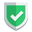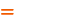我们相信：世界是美好的，你是我也是。平行空间的世界里面，不同版本的生活也在继续...

## 第一种方式，%

``````name = "苏南大叔"
print("我是 %s"%name)``````
``````me = ("苏南大叔", 18)
print("我是 %s,今年%s岁" % me)``````

``````import numpy as np
x = np.arange(0, 3)
y = x * x
for xy in zip(x, y):
print(xy, type(xy))
print("坐标为(%s,%s)" % xy)``````

## 第二种方式，format

``````print("我是{}，今年{}岁".format("苏南大叔", 18))
print("喜欢{}和{}".format("小猫", "小狗", "小动物"))
print("住在{1},{0}".format("北京", "双桥"))
print("我在{city}的{university}上学".format(city="北京", university="北京大学"))
print("{university}在{0}市{1}".format("北京", "海淀区", university="北京大学"))
print("{university}在{}市{}".format("北京", "海淀区", university="北京大学"))``````

``````me = ["苏南大叔", "newsn.net"]
print("姓名:{0},网站:{0}".format(me))
# 姓名:苏南大叔,网站:newsn.net``````

## 相似的参数

``````print("我是%s,正在%s" % ("苏南大叔", "写文章"))             # 我是苏南大叔,正在写文章
print("我是{:s},正在{:s}".format("苏南大叔", "写文章"))     # 我是苏南大叔,正在写文章``````
``````print("宽度9,2位小数,左补0：%09.2f" % 3.1415926)            # 宽度9,2位小数,左补0：000003.14
print("宽度9,2位小数,左补0：{:09.2f}".format(3.1415926))    # 宽度9,2位小数,左补0：000003.14``````

## 结束语如果本文对您有帮助，或者节约了您的时间，欢迎打赏瓶饮料，建立下友谊关系。本博客不欢迎：各种镜像采集行为。请尊重原创文章内容，转载请保留作者链接。【福利】 腾讯云最新爆款活动！1核2G云服务器首年50元！【源码】本文代码片段及相关软件，请点此获取更多信息【绝密】秘籍文章入口，仅传授于有缘之人python